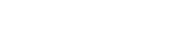# Category: Differentiation## Arc length formula

Today we are going to find the formula that allows us to calculate the arc length given a function f(x). This is quite intuitive, so you should have no problem!… Read more Arc length formula## Integral of sin(x)/x from 0 to infinity | A satisfying solution

Today we have a tough integral: not only is this a special integral (the sine integral Si(x)), but it also goes from 0 to infinity! Because of the first characteristic,… Read more Integral of sin(x)/x from 0 to infinity | A satisfying solution## Half derivative of x

The derivative of x is easy, it’s simply 1; the second derivative of x is the derivative of 1, which is 0; but what on earth is the half derivative… Read more Half derivative of x## Fundamental derivatives

What it takes to be able to calculate any derivative is to know the rules of differentiation and the fundamental derivatives, the ones you have to memorize. If you are… Read more Fundamental derivatives## The rules of differentiation

In the previous lesson we talked about differentiation in general; just as a reminder, it is a word that means “computation of a derivative”, the same way multiplication means “computation… Read more The rules of differentiation## What’s differentiation?

In my first lesson I talked about integration: in a nutshell, it’s the mathematical operation used to calculate the area under a curve of function f(x). The integral of a… Read more What’s differentiation?

{{#pages}} {{/pages}}
%%footer%%